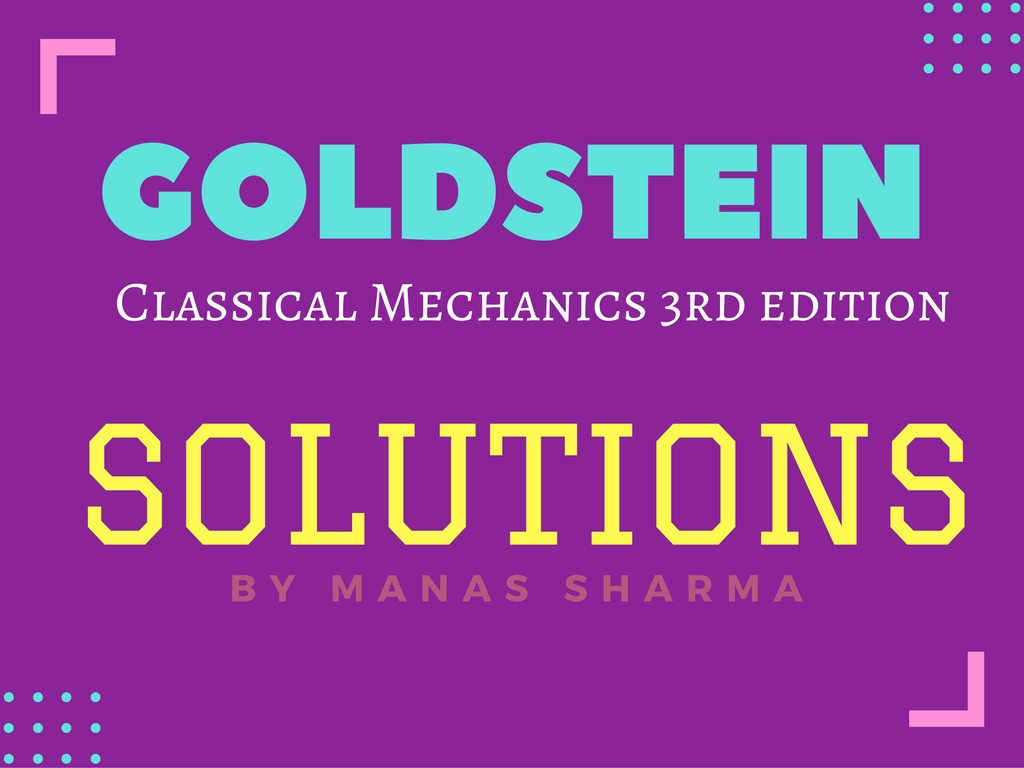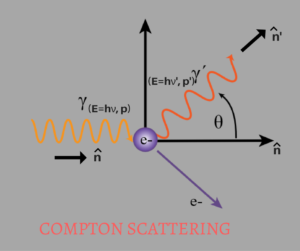# Goldstein – CHAPTER 7 [SOLUTIONS]Book: Classical Mechanics 3rd Edition
Author(s): Herbert Goldstein, Charles P. Poole, John L. Safko

I have tried solving some of the problems of the Chapter 9 of Goldstein Classical mechanics.
I have also embedded the pdf below as well as posted them in this blog post.

Goldstein Chapter 7 Solutions

Chapter 7 – The Classical Mechanics of the Special Theory of Relativity.

EXERCISES:

7.13. Show by direct multiplication of the vector form of the Lorentz transformation equations(Eq.7.9), that$r'^2-c^2t'^2=r^2-c^2t^2.$
Sol. 7.13.
From Eq.7.9. of Goldstein we have,$\boxed{\mathbf{r'}=\mathbf{r}+\frac{(\boldsymbol{\beta.}\mathbf{r})\boldsymbol{\beta}(\gamma-1))}{\beta^2}-\boldsymbol{\beta}\gamma ct}$$\implies r'^2=\mathbf{r'.r'}$$\implies r'^2=\mathbf{r.r}+\left[\frac{(\boldsymbol{\beta.}\mathbf{r})\boldsymbol{\beta}(\gamma-1)}{\beta^2} \right ]^2+\gamma^2c^2t^2\boldsymbol{\beta.\beta}+2\frac{(\boldsymbol{\beta.}\mathbf{r})(\gamma-1)}{\beta^2}\mathbf{r.}\boldsymbol{\beta}-2\frac{(\boldsymbol{\beta.}\mathbf{r})(\gamma-1)(\gamma ct)}{\beta^2}\boldsymbol{\beta.\beta} -2\gamma ct\mathbf{r.}\boldsymbol{\beta }$$\implies r'^2=r^2+\frac{(\boldsymbol{\beta.}\mathbf{r})^2(\gamma-1)^2}{\beta^2}+\gamma^2c^2t^2\beta^2+2(\boldsymbol{\beta.}\mathbf{r})^2\frac{(\gamma-1)}{\beta^2}-2(\boldsymbol{\beta.}\mathbf{r})(\gamma-1)(\gamma ct) -2\gamma ct\boldsymbol{\beta. }\mathbf{r.}$$\implies r'^2=r^2+\frac{(\boldsymbol{\beta.}\mathbf{r})^2(\gamma-1)^2}{\beta^2}+\gamma^2c^2t^2\beta^2+2(\boldsymbol{\beta.}\mathbf{r})^2\frac{(\gamma-1)}{\beta^2}-2(\boldsymbol{\beta.}\mathbf{r})\gamma^2 ct$$\implies r'^2=r^2+(\boldsymbol{\beta.}\mathbf{r})^2 \left[ \frac{(\gamma-1)^2}{\beta^2}+2\frac{(\gamma-1)}{\beta^2}\right]+\gamma^2c^2t^2\beta^2-2(\boldsymbol{\beta.}\mathbf{r})\gamma^2 ct .....(i)$

Also from Eq.7.9 of Goldstein we have,$\boxed{ct'=\gamma(ct-\boldsymbol{\beta.}\mathbf{r})}$$\implies (ct')^2=\gamma^2(ct-\boldsymbol{\beta.}\mathbf{r})^2$$\implies (ct')^2=\gamma^2\left(c^2t^2+(\boldsymbol{\beta.}\mathbf{r})^2-2ct\boldsymbol{\beta}\mathbf{.r}\right)$$\implies (ct')^2=\gamma^2c^2t^2+\gamma^2\left(\boldsymbol{\beta}\mathbf{.r}\right)^2-2\gamma^2ct(\boldsymbol{\beta}\mathbf{.r}) .....(ii)$

From eq(i) and (ii) we have,$r'^2-c^2t'^2=r^2+(\boldsymbol{\beta.}\mathbf{r})^2 \left[ \frac{(\gamma-1)^2}{\beta^2}+2\frac{(\gamma-1)}{\beta^2}-\gamma^2\right]+\gamma^2c^2t^2(\beta^2-1)-2(\boldsymbol{\beta.}\mathbf{r})\gamma^2 ct +2\gamma^2ct(\boldsymbol{\beta}\mathbf{.r})$$\because \left[ \frac{(\gamma-1)^2}{\beta^2}+2\frac{(\gamma-1)}{\beta^2}-\gamma^2\right]=0$$\implies r'^2-c^2t'^2=r^2+\gamma^2c^2t^2(\beta^2-1)$
Now, we know that$\gamma^2=\frac{1}{1-\beta^2}$$\therefore \boxed{r'^2-c^2t'^2=r^2-c^2t^2 }$

Hence Proved.

7.14. Calculate the length of a rod of rest length 2m, moving at 0.73c as observed by an observer at rest. Also, compute at what speed the length in motion of the rod will be half the rest length.
Sol. 7.14.

We have,
Rest length,$l_0=2m$
and$\beta=0.73$

The observed length ‘l’ is related to the rest length as,$l=\frac{l_0}{\gamma}$$\implies l=l_0\sqrt{1-\beta^2}=2\sqrt{1-(0.73)^2}$$\implies l=2\sqrt{1-0.5329}$$\implies l=2\sqrt{0.4671}$$\implies l=2(0.6834)$$\implies l=1.3668m$

Now, we are asked to find the speed(or β) for which length is half the rest length,
i.e.$\frac{l}{l_0}=\frac{1}{2}=\frac{1}{\gamma}$$\implies \gamma=2$$\implies \frac{1}{\sqrt{1-\beta^2}}=2$$\implies 1=4(1-\beta^2)$$\implies 4\beta^2=3$$\implies \beta=\sqrt{\frac{3}{4}}$$\implies \boxed{v= \sqrt{\frac{3}{4}}c}$

7.18. Calculate the mass of an electron moving at 0.84c. Also, comment whether the electron can be accelerated to the velocity of light. (Rest Mass of an electron is 9.1×10^-31kg.)
Sol.7.18.

We are given,
Rest mass:$m_0=9.1 \times 10^{-31}$
and$\beta= 0.84$
Relativistic mass, ‘m’, is given as$m=\gamma m_0$
where m0 is the rest mass.$\implies m=\frac{1}{\sqrt{1-\beta^2}}m_0$$\implies m=\frac{1}{\sqrt{1-(0.84)^2}}m_0$$\implies m=\frac{1}{\sqrt{1-0.7056}}m_0$$\implies m=\frac{1}{\sqrt{0.2944}}m_0$$\implies \boxed{m=1.8430m_0}$

7.19. A meson of mass mπ at rest disintegrates into a meson of mass mμ and a neutrino of effectively zero mass. Show that the kinetic energy of the μ meson is$T=\frac{(m_\pi-m_\mu)^2}{2m_\pi}c^2$
Sol.7.19.
We are given the following process:$\pi\longrightarrow \mu+\nu$

Four-Momentum of a particle is given by,$P^\mu=\left[\frac{E}{c},\mathbf{p}\right]$
where E is the total energy of the particle,
p is the momentum(vector) of the particle.

Using the above formula,
The Four-Momenta of the particles are as follows:
For pion:$P^\alpha_\pi=\left[\frac{E_\pi}{c},\mathbf{0}\right]=\left[m_\pi c,0\right]$
For muon:$P^\alpha_\mu=\left[\frac{E_\mu}{c},\mathbf{p_\mu}\right]$
For neutrino:$P^\alpha_\nu=\left[\frac{E_\nu}{c},\mathbf{p_\nu}\right]$

Conservation of energy and momentum gives:$P^\alpha_\pi=P^\alpha_\mu + P^\alpha_\nu$$\implies P^\alpha_\pi-P^\alpha_\mu = P^\alpha_\nu$$\implies \left\|P^\alpha_\pi-P^\alpha_\mu \right\| = \left\|P^\alpha_\nu\right\|$$\implies \left(P^\alpha_\pi -P^\alpha_\mu \right)^2 = \left(P^\alpha_\nu \right)^2$$\implies P^\alpha_\pi P_{\pi \alpha}+P^\alpha_\mu P_{\mu \alpha}-2P^\alpha_\pi P_{\mu \alpha}=P^\alpha_\nu P_{\nu \alpha}$$\implies m^2_\pi c^2 + m^2_\mu c^2-2\left[m_\pi c\frac{\left(E_\mu\right)}{c}+0\right]=0$$\implies 2m_\pi E_\mu = m^2_\pi c^2+m^2_\mu c^2$$\implies E_\mu=\frac{(m^2_\pi + m^2_\mu)c^2}{2m_\pi}$
The above is the total energy(K.E.+Rest Mass Energy) of the muon.
We need to find out the Kinetic Energy of muon, which is the total energy minus the rest mass energy,$T_\mu=E_\mu-m_\mu c^2$$\implies T_\mu=\frac{m^2_\pi c^2+m^2_\mu c^2-2m_\pi m_\mu c^2}{2m_\pi}$$\implies \boxed{T_\mu=\frac{(m_\pi -m_\mu)^2c^2}{2m_\pi}}$
Hence Proved.

7.20. Calculate the mean life of a pion travelling at 0.8c. (Proper mean lifetime for the pion is 28 nano second.)
Sol.7.20.

The proper time(τ) is related to the dilated time(t) as:$\tau=\frac{t}{\gamma}$$\implies t=\gamma \tau$$\implies t=\frac{1}{\sqrt{1-(0.8)^2}}\tau$$\implies t=\frac{1}{\sqrt{0.36}}\tau$$\implies t=\frac{1}{0.6}\tau$$\implies \boxed{t=1.6667\tau}$
Therefore the mean lifetime of the travelling pion is 1.6667 times that of the pion at rest.

7.21. A photon may be described classically as a particle of zero mass possessing nevertheless a momentum h/λ = hν/c, and therefore a kinetic energy hν. If the photon collides with an electron of mass m at rest, it will be scattered at some angle θ with a new energy hν’. Show that the changes in energy is related to the scattering angle by the formula$\lambda'-\lambda=2\lambda_c\sin^2\frac{\theta}{2}$
where λc=h/mc, is known as the Compton wavelength. Show also that the kinetic of the recoil motion of the electron is$T_{e^-} = h\nu \frac{2\left(\frac{\lambda_c}{\lambda}\right)\sin^2\frac{\theta}{2}}{1+2\left(\frac{\lambda_c}{\lambda}\right)\sin^2\frac{\theta}{2}}$
Sol.7.21.The above diagram shows the process described in the problem. As you can see it is in fact, the famous, ‘Compton Scattering’.

We will solve this problem using 4-momenta.
The four momenta of the particles before and after the collision are as follows:
Photon, before collision, moves with energy hν and momentum p in the direction n, therefore its 4-momentum is:$P^\mu_\gamma=\left[ \frac{E}{c}, \mathbf{p} \right]=\left[ \frac{h\nu}{c},\frac{h\nu}{c}\hat{\textup{n}} \right]$
Photon, after collision, moves with energy hν’ and momentum p’ in the n’ direction, therefore its 4-momentum is:$P^\mu_{\gamma'}=\left[ \frac{E'}{c}, \mathbf{p'} \right]=\left[ \frac{h\nu'}{c},\frac{h\nu'}{c}\hat{\textup{n}'} \right]$
Electron, before collision, is at rest(hence momentum=0) and has rest mass energy mec^2, therefore its 4-momentum is:$P^\mu_{e^-}=\left[ \frac{E_{e^-}}{c},0\right]=\left[m_ec,0\right]$
Electron, after collision, moves with energy E’e and momentum p’e, therefore its 4-momentum is:$P^\mu_{{e^-}'}=\left[ \frac{E_{e^-}'}{c}, \mathbf{p'_{e^-}}\right]$

From the conservation of Energy and Momentum we have,$P^\mu_\gamma + P^\mu_{e^-} = P^\mu_{\gamma'} + P^\mu_{{e^-}'} \hspace{1cm}\textup{(i)}$$\implies P^\mu_\gamma - P^\mu_{\gamma'} + P^\mu_{e^-} = P^\mu_{{e^-}'}$$\implies \left\|P^\mu_\gamma - P^\mu_{\gamma'} + P^\mu_{e^-} \right\|= \left\|P^\mu_{{e^-}'}\right\|$$P^\mu_\gamma P_{\mu\gamma } + P^\mu_{\gamma'}P_{\mu\gamma'} + P^\mu_{e^-}P_{\mu e^-}-2P^\mu_\gamma P_{\mu\gamma'}-2P^\mu_{\gamma'}P_{\mu e^-} +2P^\mu_\gamma P_{\mu e^-} = P^\mu_{{e^-}'}P_{\mu{e^-}'}$$\implies 0+0+m_e^2c^2-2\left[ \frac{h\nu}{c}\frac{h\nu'}{c}+ \frac{h\nu}{c}\frac{h\nu'}{c}\hat{\textup{n}}.\hat{\textup{n}}' \right] -2\left[ \frac{h\nu'}{c}m_ec + 0 \right] + 2\left[ \frac{h\nu}{c}m_ec + 0 \right] = m^2_ec^2$$\implies m_eh(\nu-\nu')=\frac{h\nu h\nu'}{c^2}(1+\cos\theta)$$\implies \nu-\nu'= \frac{h\nu h\nu'}{m_ec^2}(1+\cos\theta)$$\implies \frac{c}{\lambda} - \frac{c}{\lambda}=\frac{hc^2}{\lambda\lambda'm_ec^2}(1+\cos\theta)$$\implies \left(\frac{\lambda'-\lambda}{\lambda\lambda'}\right)c=\frac{c}{\lambda\lambda'}\left(\frac{h}{m_ec}\right)(1+\cos\theta)$$\implies \lambda'-\lambda=\lambda_c\left( 2\sin^2\frac{\theta}{2} \right)$$\implies \boxed{\lambda'-\lambda=2\lambda_c\sin^2\frac{\theta}{2}}$
——————————————————————–
Remarks:
1. If θ=0, then that isn’t much scattering, therefore λ’=λ, as expected.
2. If θ=π (that is backward scattering) and additionally λ’ << h/mc (that is, mc2<<hc/λ=Eγ), then λ’=2h/mc, so$E_\gamma=\frac{hc}{\lambda'}\approx \frac{hc}{\frac{2h}{mc}} = \frac{1}{2}mc^2$
Therefore, the photon bounces back with an essentially ﬁxed E’γ, independent of the initial (as long as is large enough). This isn’t all that obvious.
——————————————————————–
Now, for the second part of the question, rearranging eq(i), we get$P^\mu_\gamma - P^\mu_{\gamma'} = P^\mu_{{e^-}'} - P^\mu_{e^-}$$\implies \left(P^\mu_\gamma - P^\mu_{\gamma'} \right)^2= \left(P^\mu_{{e^-}'} - P^\mu_{e^-}\right)^2$$\implies P^\mu_\gamma P_{\mu\gamma} + P^\mu_{\gamma'}P_{\mu\gamma'}-2P^\mu_\gamma P_{\mu\gamma'}= P^\mu_{{e^-}'}P_{\mu {e^-}'}+P^\mu_{e^-} P_{\mu e^-} -2P^\mu_{{e^-}'}P_{\mu e^-}$$\implies 0 + 0 + -2\left[ \frac{h\nu}{c}\frac{h\nu'}{c} + \frac{h\nu}{c}\frac{h\nu'}{c}\cos\theta \right] = m^2_ec^2 + m^2_ec^2 - 2\left[ E'_{e^-}m_e + 0 \right]$$\implies m^2_ec^2 + \frac{h\nu h\nu'}{c^2}(1+\cos\theta) = E'_{e^-}m_e$$\implies m_ec^2 +\frac{h\nu h\nu'}{m_ec^2}\left( 2\sin^2\frac{\theta}{2} \right) = E'_{e^-}$$\implies E'_{e^-} = m_ec^2 + 2\frac{h\nu \nu'}{c}\left(\frac{h}{m_ec}\right)\sin^2\frac{\theta}{2}$
This is the total energy of the recoil electron.
We are interested in the Kinetic Energy, which is the Total Energy-Rest mass Energy:$T_{e^-}=E'_{e^-}-m_ec^2$$\implies T_{e^-}= 2\frac{h\nu}{\lambda'}\lambda_c \sin^2\frac{\theta}{2}$
using the relation derived in the first part of the question,$\lambda'=\lambda + 2\lambda_c\sin^2\frac{\theta}{2}$
Plugging this value of λ’ in the expression of Kinetic Energy,$\implies T_{e^-}=h\nu \frac{2\lambda_c \sin^2\frac{\theta}{2}}{\lambda+2\lambda_c \sin^2\frac{\theta}{2}}$$\implies \boxed{T_{e^-} = h\nu \frac{2\left(\frac{\lambda_c}{\lambda}\right)\sin^2\frac{\theta}{2}}{1+2\left(\frac{\lambda_c}{\lambda}\right)\sin^2\frac{\theta}{2}} }$
which is the required result.

Links you might find useful:
*Compton Scattering
*Online Latex Editor

If you have any doubts/question/suggestions regarding the solutions, just hit me up in comments section down below.[wpedon id="7041" align="center"]